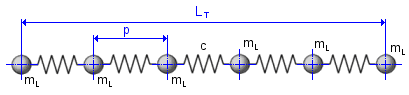# Lessons from the Coupled Oscillator

In studying solid state physics, one of the first problems encountered is that of phonons. In the usual textbooks (such as Ashcroft and Mermin or Kittel), the physics is buried underneath formalism. Here is my attempt to explain the physics, while just quoting the main mathematical results. For the simple mass-spring oscillator system pictured below, we get the following equation of motion and oscillation frequency:Simple harmonic oscillator$\ddot{x} = -\omega^2x$

and$\omega^2 = \frac{k}{m}$

If we couple two harmonic oscillators, such as in the situation below, we get two normal modes that obey the equations of motion identical to the single-oscillator case.

The equations of motion for the normal modes are:$\ddot{\eta_1} = -\omega^2_1\eta_1$      and$\ddot{\eta_2} = -\omega^2_2\eta_2$,

where$\omega_1^2 = \frac{k+2\kappa}{m}$

and$\omega_2^2 = \frac{k}{m}$.

I should also mention that$\eta_1 = x_1 - x_2$$\eta_2 = x_1 + x_2$. The normal modes are pictured below, consisting of a symmetric and antisymmetric oscillation:Symmetric normal mode

The surprising thing about the equations for the normal modes is that they look exactly like the equations for two decoupled and independent harmonic oscillators. Any motion of the oscillators can therefore be written as a linear combination of the normal modes. When looking back at such results, it seems trivial — but I’m sure to whoever first solved this problem, the result was probably unexpected and profound.

Now, let us briefly discuss the quantum case. If we have a single harmonic oscillator, we get that the Hamiltonian is:$H = \hbar\omega (a^\dagger a +1/2)$

If we have many harmonic oscillators coupled together as pictured below, one would probably guess in light of the classical case that one could obtain the normal modes similarly.Harmonic Chain

One would probably then naively guess that the Hamiltonian could be decoupled into many seemingly independent oscillators:$H = \sum_k\hbar\omega_k (a^\dagger_k a _k+1/2)$

This intuition is exactly correct and this is indeed the Hamiltonian describing phonons, the normal modes of a lattice. The startling conclusion in the quantum mechanical case, though, is that the equations lend themselves to a quasiparticle description — but I wish to speak about quasiparticles another day. Many ideas in quantum mechanics, such as Anderson localization, are general wave phenomena and can be seen in classical systems as well. Studying and visualizing classical waves can therefore still yield interesting insights into quantum mechanics.